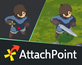# AttachPoint | Animate 2D Points

Create attach points on your sprites for sticking objects! · By Gurpreet Singh Matharoo

# Code for image_angle/x/yscale?

A topic by lorewap3 created Feb 22, 2020 Views: 132 Replies: 3
Viewing posts 1 to 4

Hey Matharoo,

I'm really enjoying the tool, its immensely helpful! I was hoping you could help me out with a little implementation, though.

The attach points work fine with a character with image_x/yscale = 1 and image_angle = 0, but in betweens I'm having trouble figuring out the correct algorithm.

I got the 4 combinations of image_xscale -1 / 1 and image_yscale -1 /1 to work right using 4 if statements, but adding image_angle to the mix has been difficult. I'm thinking I need to remove image_yscale from the mix and just use image_xscale and image_angle. I would appreciate any thoughts you might have on the subject. Or perhaps you wouldn't mind sharing the code that you use?

Here's a sample of the code I'm using. The attached point is a particle emitter, so emitterX/Y/Direction are the values I use after recalculating from the original attach points.

var _curr_attach_point = _attach_points[_curr_index];

var _offset_x = _curr_attach_point;
var _offset_y = _curr_attach_point;

var _length = sqrt(sqr(_offset_x) + sqr(_offset_y));

var _adj_x = lengthdir_x(_length,image_angle);
var _adj_y = lengthdir_y(_length,image_angle);

// Normal direction
if (image_xscale > 0 && image_yscale > 0) {
emitterX = x + _adj_x;
emitterY = y + _adj_y;
emitterDirection =  _curr_attach_point;

// Flipped horizontally
} else if (image_xscale < 0 && image_yscale > 0) {
emitterX = x - _adj_x;
emitterY = y + _adj_y;
emitterDirection = 180 - _curr_attach_point;

// Flipped vertically
} else if (image_xscale > 0 && image_yscale < 0) {
emitterX = x + _adj_x;
emitterY = y - _adj_y;
emitterDirection = 180 - _curr_attach_point;

// Flipped horizontally and vertically
} else if (image_xscale < 0 && image_yscale < 0) {
emitterX = x - _adj_x;
emitterY = y - _adj_y;
emitterDirection = 180 + _curr_attach_point;
}

emitterDirection += image_angle;

I appreciate any help you can give! I'm really liking your tool! As well as your tutorials!

Will

Developer

Hey Will,

Could you email me your project, to gurpreetsingh793@gmail.com? It would be quicker if I could fix the code in the project itself and then explain what I did.

Cheers

Hey Matharoo,

Sorry I didn't respond until now. It was going to be difficult to send you the project as it currently is, I load alot of json files from the filesystem and the code that calculates the attach_point is buried below a good number of inheritance that would've taken just as long to explain hah. But I figured it out. I just had to think it through procedurally. I have sprites that I flip the image_xscale , image_yscale, and image_angle after that so I just needed to process it in order.

Here's the code in case you are interested!

In essence I get the current image_index, then calculate what the attach_point would be considering ONLY image_xscale and image_yscale, which isn't too bad. Then after that's all done I calculate the image_angle. What was throwing me off is that once you flip the image on the x or y axis, it also flips the attach_point direction the opposite way. So the code I had was already correct, it just wasn't the result I expected.

Thank you for replying so quickly though, I appreciate your willingness to help! I appreciate your videos!

var _curr_index = floor(image_index);

if (_curr_index >= array_length_1d(_attach_points)) {
_curr_index = array_length_1d(_attach_points) - 1;
} else if (_curr_index < 0) {
_curr_index = 0;
}

var _curr_attach_point = _attach_points[_curr_index];

_offset_x = _curr_attach_point;
_offset_y = _curr_attach_point;
_attach_point_direction = _curr_attach_point;

// **************************************
// **************************************
#region Calculates x/y coordinate and direction of attach point assuming image_angle = 0
// Normal direction
if (image_xscale > 0 && image_yscale > 0) {
_adj_offset_x = _offset_x;
_adj_offset_y = _offset_y;
_adj_attach_point_direction = _attach_point_direction;

// Flipped horizontally
} else if (image_xscale < 0 && image_yscale > 0) {
_adj_offset_x = _offset_x * -1;
_adj_offset_y = _offset_y;
_adj_attach_point_direction = 180 - _attach_point_direction;

} else if (image_xscale > 0 && image_yscale < 0) {
_adj_offset_x = _offset_x;
_adj_offset_y = _offset_y * -1;
_adj_attach_point_direction = 360 - _attach_point_direction;

} else if (image_xscale < 0 && image_yscale < 0) {
_adj_offset_x = _offset_x * -1;
_adj_offset_y = _offset_y * -1;
_adj_attach_point_direction = 180 + _attach_point_direction;
}

_adj_attach_point_direction = wrap(_adj_attach_point_direction, 0, 359);
#endregion
// **************************************
// **************************************

// **************************************
// **************************************
#region Calculate x/y/direction taking image_angle into account
if (image_angle != 0) {
// Gets angle of the straight line drawn from center of player object to attach point
_attach_point_direction_from_center = point_direction(0,0,_adj_offset_x, _adj_offset_y);

// Once starting direction is determined, calculate adjusted based on image_angle
_img_angle_adj_direction_first = _attach_point_direction_from_center + image_angle;

// Get length of line from center of player object to attach point
_length = sqrt(sqr(_adj_offset_x) + sqr(_adj_offset_y));

// Gets new x/y coordinates of new attach_point angle when you add in image_angle
_img_angle_adj_x = lengthdir_x(_length, _img_angle_adj_direction_first);
_img_angle_adj_y = lengthdir_y(_length, _img_angle_adj_direction_first);

// Finally, add direction of actual attach point to new calculated direction
//_img_angle_adj_direction = _img_angle_adj_direction_first + _adj_attach_point_direction;
_img_angle_adj_direction = _adj_attach_point_direction + image_angle;
} else {
_attach_point_direction_from_center = "N/A";
_img_angle_adj_direction_first = "N/A";
_length = "N/A";

_img_angle_adj_x = _adj_offset_x;
_img_angle_adj_y = _adj_offset_y;
_img_angle_adj_direction = _adj_attach_point_direction;
}
_img_angle_adj_direction = wrap(_img_angle_adj_direction, 0, 359);
#endregion
// **************************************
// **************************************

emitterX = x + _img_angle_adj_x;
emitterY = y + _img_angle_adj_y;
emitterDirection = _img_angle_adj_direction;

Developer

Awesome that you got it to work! 👍